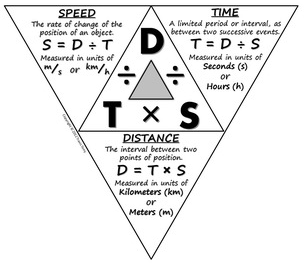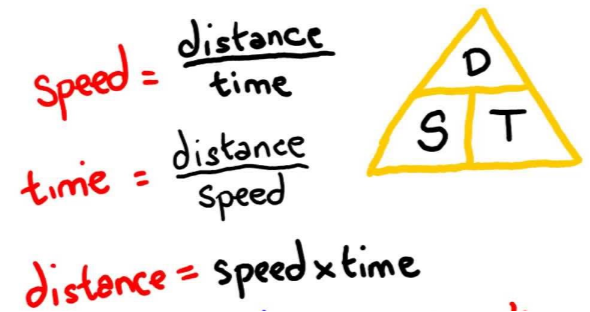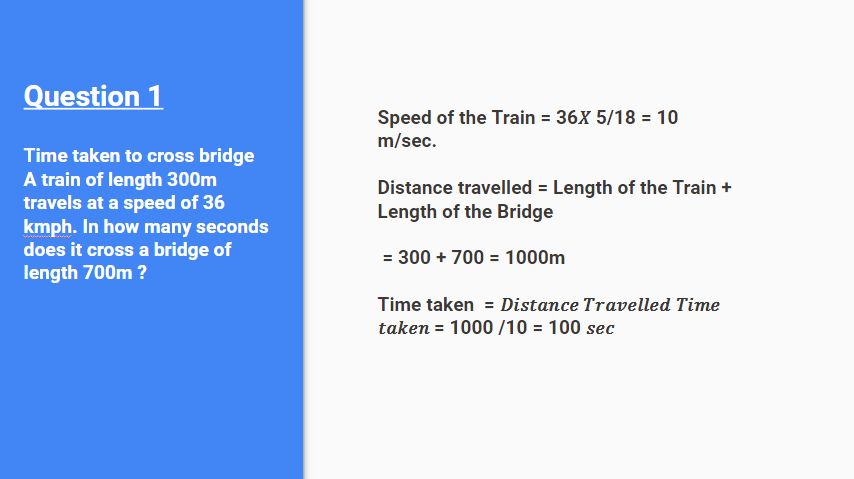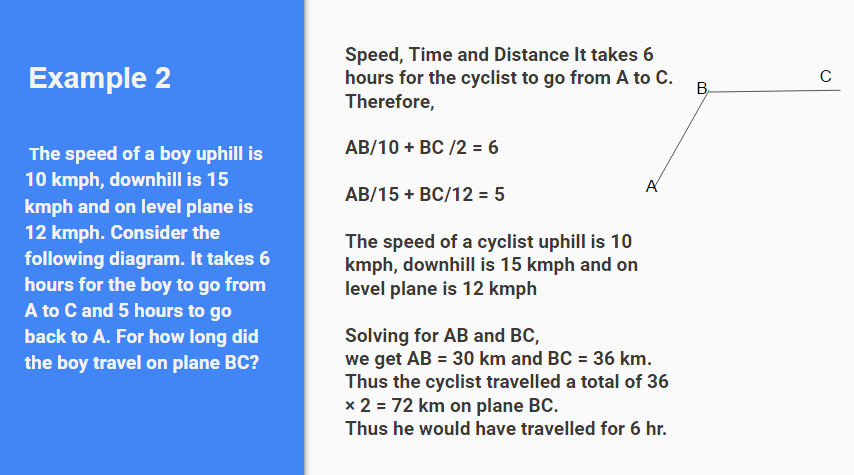Share It On :

Time & distanceTricks

• To convert from

km/hr to m/sec = Multiply by 𝟓/𝟏𝟖

A km/hr = A x 5/18  m/s

• To convert from

m/sec to Km/hr = Multiply by 𝟏𝟖/𝟓

B m/ s = B x 18/ 5km/hr

• If a car covers a certain distance at A kmph and an equal distance at B kmph, the average Speed of the whole journey =

2AB / A+B

• Speed and time are inversely proportional (when distance> is constant)

• If the ratio of the speeds of A and B is

a : b,

• Then the ratio of the times taken by them to cover the same distance is

b : a

ExamplesHomework

1. In a race of 200 metres, Y can give a start of 10 metres to X, and Z can give a start of 20 metres to Y. The start that Z can give to X, in the same race, is

(a) 30 m

(b) 25 m

(c) 29 m

(d) 27 m

2. A BUS, 240 m long crosses a man walking along the line in opposite direction at the rate of 3 kmph in 10 seconds. The speed of the BUS is

(a) 63 kmph

(b) 75 kmph

(d) 83.4 kmph

(d) 86.4 kmph

3. A train 300 metres long is running at a speed of 25 metres per second. It will cross a RAILWAY bridge of 200 metres in

(a) 5 seconds

(b) 10 seconds

(c) 20 seconds

(d) 25 seconds

4. A CAR moving at a speed of 45 km/hr overtakes a BUS 150 metres ahead going in the same direction in 30 seconds. The speed of the BUS is

(a) 27 km/hr

(b) 24 km/hr

(c) 25 km/hr

(d) 28 km/hr

5. A BOY crosses a road 250 metres wide in 75 seconds. His speed in km/hr is:

(a) 10

(b) 12

(c) 12.5

(d) 15

1.C

2. C

3. C

4. A

5. B